Learn data science and machine learning by building real-world projects on Jovian
. 20 weeks part-time, 7 courses, 12 assignments, 4 projects, and 6 months of career support.

## Data Visualization using Python, Matplotlib and Seaborn#### Part 8 of "Data Analysis with Python: Zero to Pandas"

This tutorial series is a beginner-friendly introduction to programming and data analysis using the Python programming language. These tutorials take a practical and coding-focused approach. The best way to learn the material is to execute the code and experiment with it yourself. Check out the full series here:

This tutorial covers the following topics:

• Creating and customizing line charts using Matplotlib
• Visualizing relationships between two or more variables using scatter plots
• Studying distributions of variables using histograms & bar charts to
• Visualizing two-dimensional data using heatmaps
• Displaying images using Matplotlib's `plt.imshow`
• Plotting multiple Matplotlib and Seaborn charts in a grid

#### How to run the code

This tutorial is an executable Jupyter notebook hosted on Jovian. You can run this tutorial and experiment with the code examples in a couple of ways: using free online resources (recommended) or on your computer.

##### Option 1: Running using free online resources (1-click, recommended)

The easiest way to start executing the code is to click the Run button at the top of this page and select Run on Binder. You can also select "Run on Colab" or "Run on Kaggle", but you'll need to create an account on Google Colab or Kaggle to use these platforms.

##### Option 2: Running on your computer locally

To run the code on your computer locally, you'll need to set up Python, download the notebook and install the required libraries. We recommend using the Conda distribution of Python. Click the Run button at the top of this page, select the Run Locally option, and follow the instructions.

Jupyter Notebooks: This tutorial is a Jupyter notebook - a document made of cells. Each cell can contain code written in Python or explanations in plain English. You can execute code cells and view the results, e.g., numbers, messages, graphs, tables, files, etc., instantly within the notebook. Jupyter is a powerful platform for experimentation and analysis. Don't be afraid to mess around with the code & break things - you'll learn a lot by encountering and fixing errors. You can use the "Kernel > Restart & Clear Output" menu option to clear all outputs and start again from the top.

### Introduction

Data visualization is the graphic representation of data. It involves producing images that communicate relationships among the represented data to viewers. Visualizing data is an essential part of data analysis and machine learning. We'll use Python libraries Matplotlib and Seaborn to learn and apply some popular data visualization techniques. We'll use the words chart, plot, and graph interchangeably in this tutorial.

To begin, let's install and import the libraries. We'll use the `matplotlib.pyplot` module for basic plots like line & bar charts. It is often imported with the alias `plt`. We'll use the `seaborn` module for more advanced plots. It is commonly imported with the alias `sns`.

In :
``!pip install matplotlib seaborn --upgrade --quiet``
In :
``````import matplotlib.pyplot as plt
import seaborn as sns
%matplotlib inline
``````

Notice this we also include the special command `%matplotlib inline` to ensure that our plots are shown and embedded within the Jupyter notebook itself. Without this command, sometimes plots may show up in pop-up windows.

### Line Chart

The line chart is one of the simplest and most widely used data visualization techniques. A line chart displays information as a series of data points or markers connected by straight lines. You can customize the shape, size, color, and other aesthetic elements of the lines and markers for better visual clarity.

Here's a Python list showing the yield of apples (tons per hectare) over six years in an imaginary country called Kanto.

In :
``yield_apples = [0.895, 0.91, 0.919, 0.926, 0.929, 0.931]``

We can visualize how the yield of apples changes over time using a line chart. To draw a line chart, we can use the `plt.plot` function.

In :
``plt.plot(yield_apples)``
Out:
``[<matplotlib.lines.Line2D at 0x7f90047a8520>]``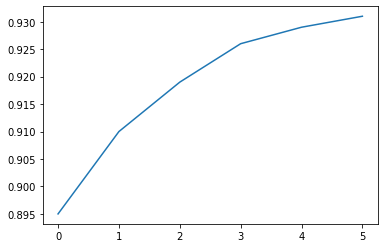Calling the `plt.plot` function draws the line chart as expected. It also returns a list of plots drawn `[<matplotlib.lines.Line2D at 0x7ff70aa20760>]`, shown within the output. We can include a semicolon (`;`) at the end of the last statement in the cell to avoiding showing the output and display just the graph.

In :
``plt.plot(yield_apples);``Let's enhance this plot step-by-step to make it more informative and beautiful.

#### Customizing the X-axis

The X-axis of the plot currently shows list element indexes 0 to 5. The plot would be more informative if we could display the year for which we're plotting the data. We can do this by two arguments `plt.plot`.

In :
``````years = [2010, 2011, 2012, 2013, 2014, 2015]
yield_apples = [0.895, 0.91, 0.919, 0.926, 0.929, 0.931]``````
In :
``plt.plot(years, yield_apples)``
Out:
``[<matplotlib.lines.Line2D at 0x7f90051fcfa0>]``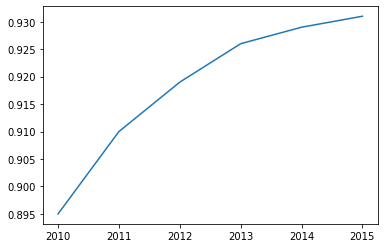#### Axis Labels

We can add labels to the axes to show what each axis represents using the `plt.xlabel` and `plt.ylabel` methods.

In :
``````plt.plot(years, yield_apples)
plt.xlabel('Year')
plt.ylabel('Yield (tons per hectare)');``````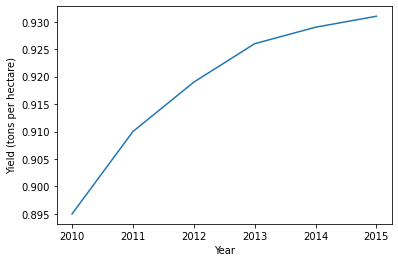#### Plotting Multiple Lines

You can invoke the `plt.plot` function once for each line to plot multiple lines in the same graph. Let's compare the yields of apples vs. oranges in Kanto.

In :
``````years = range(2000, 2012)
apples = [0.895, 0.91, 0.919, 0.926, 0.929, 0.931, 0.934, 0.936, 0.937, 0.9375, 0.9372, 0.939]
oranges = [0.962, 0.941, 0.930, 0.923, 0.918, 0.908, 0.907, 0.904, 0.901, 0.898, 0.9, 0.896, ]``````
In :
``````plt.plot(years, apples)
plt.plot(years, oranges)
plt.xlabel('Year')
plt.ylabel('Yield (tons per hectare)');``````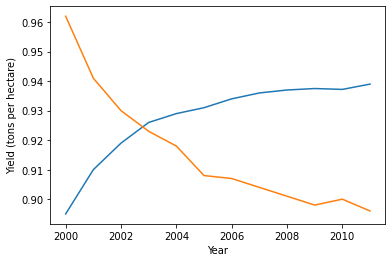#### Chart Title and Legend

To differentiate between multiple lines, we can include a legend within the graph using the `plt.legend` function. We can also set a title for the chart using the `plt.title` function.

In :
``````plt.plot(years, apples)
plt.plot(years, oranges)

plt.xlabel('Year')
plt.ylabel('Yield (tons per hectare)')

plt.title("Crop Yields in Kanto")
plt.legend(['Apples', 'Oranges']);``````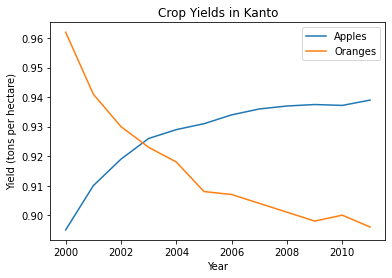#### Line Markers

We can also show markers for the data points on each line using the `marker` argument of `plt.plot`. Matplotlib provides many different markers, like a circle, cross, square, diamond, etc. You can find the full list of marker types here: https://matplotlib.org/3.1.1/api/markers_api.html .

In :
``````plt.plot(years, apples, marker='o')
plt.plot(years, oranges, marker='x')

plt.xlabel('Year')
plt.ylabel('Yield (tons per hectare)')

plt.title("Crop Yields in Kanto")
plt.legend(['Apples', 'Oranges']);``````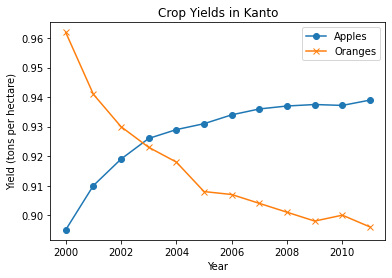#### Styling Lines and Markers

The `plt.plot` function supports many arguments for styling lines and markers:

• `color` or `c`: Set the color of the line (supported colors)
• `linestyle` or `ls`: Choose between a solid or dashed line
• `linewidth` or `lw`: Set the width of a line
• `markersize` or `ms`: Set the size of markers
• `markeredgecolor` or `mec`: Set the edge color for markers
• `markeredgewidth` or `mew`: Set the edge width for markers
• `markerfacecolor` or `mfc`: Set the fill color for markers
• `alpha`: Opacity of the plot

Check out the documentation for `plt.plot` to learn more: https://matplotlib.org/api/_as_gen/matplotlib.pyplot.plot.html#matplotlib.pyplot.plot .

In :
``````plt.plot(years, apples, marker='s', c='b', ls='-', lw=2, ms=8, mew=2, mec='navy')
plt.plot(years, oranges, marker='o', c='r', ls='--', lw=3, ms=10, alpha=.5)

plt.xlabel('Year')
plt.ylabel('Yield (tons per hectare)')

plt.title("Crop Yields in Kanto")
plt.legend(['Apples', 'Oranges']);``````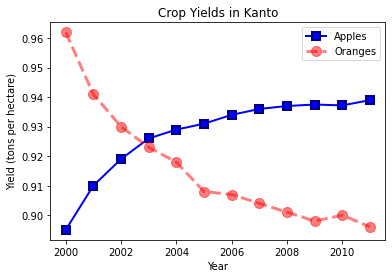The `fmt` argument provides a shorthand for specifying the marker shape, line style, and line color. It can be provided as the third argument to `plt.plot`.

``````fmt = '[marker][line][color]'
``````
In :
``````plt.plot(years, apples, 's-b')
plt.plot(years, oranges, 'o--r')

plt.xlabel('Year')
plt.ylabel('Yield (tons per hectare)')

plt.title("Crop Yields in Kanto")
plt.legend(['Apples', 'Oranges']);``````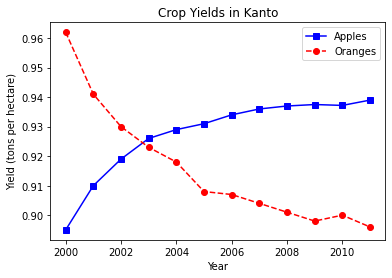If you don't specify a line style in `fmt`, only markers are drawn.

In :
``````plt.plot(years, oranges, 'or')
plt.title("Yield of Oranges (tons per hectare)");``````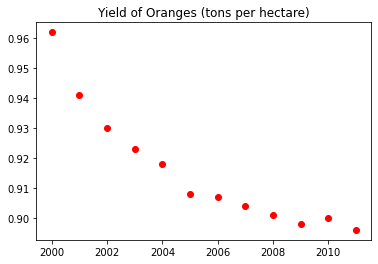#### Changing the Figure Size

You can use the `plt.figure` function to change the size of the figure.

In :
``````plt.figure(figsize=(12, 6))

plt.plot(years, oranges, 'or')
plt.title("Yield of Oranges (tons per hectare)");``````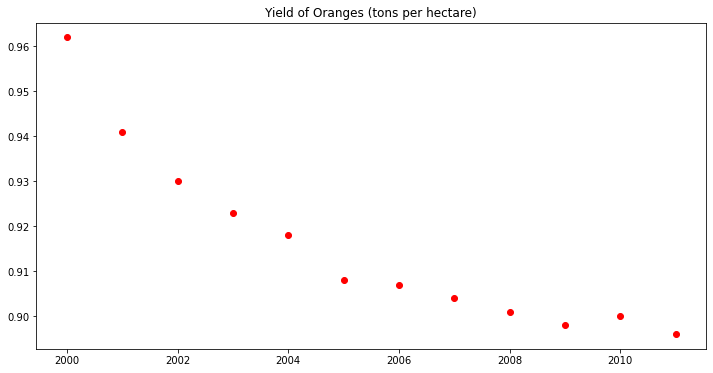#### Improving Default Styles using Seaborn

An easy way to make your charts look beautiful is to use some default styles from the Seaborn library. These can be applied globally using the `sns.set_style` function. You can see a full list of predefined styles here: https://seaborn.pydata.org/generated/seaborn.set_style.html .

In :
``sns.set_style("whitegrid")``
In :
``````plt.plot(years, apples, 's-b')
plt.plot(years, oranges, 'o--r')

plt.xlabel('Year')
plt.ylabel('Yield (tons per hectare)')

plt.title("Crop Yields in Kanto")
plt.legend(['Apples', 'Oranges']);``````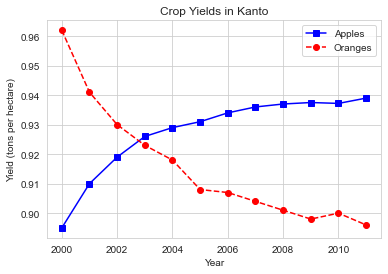In :
``sns.set_style("darkgrid")``
In :
``````plt.plot(years, apples, 's-b')
plt.plot(years, oranges, 'o--r')

plt.xlabel('Year')
plt.ylabel('Yield (tons per hectare)')

plt.title("Crop Yields in Kanto")
plt.legend(['Apples', 'Oranges']);``````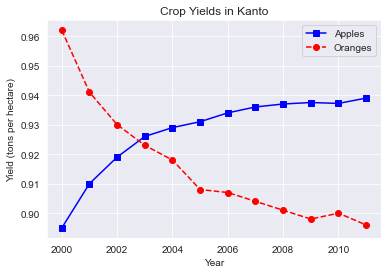In :
``````plt.plot(years, oranges, 'or')
plt.title("Yield of Oranges (tons per hectare)");``````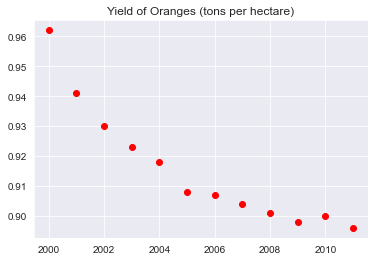You can also edit default styles directly by modifying the `matplotlib.rcParams` dictionary. Learn more: https://matplotlib.org/3.2.1/tutorials/introductory/customizing.html#matplotlib-rcparams .

In :
``import matplotlib``
In :
``````matplotlib.rcParams['font.size'] = 14
matplotlib.rcParams['figure.figsize'] = (9, 5)
matplotlib.rcParams['figure.facecolor'] = '#00000000'``````

Whether you're running this Jupyter notebook online or on your computer, it's essential to save your work from time to time. You can continue working on a saved notebook later or share it with friends and colleagues to let them execute your code. Jovian offers an easy way of saving and sharing your Jupyter notebooks online.

In :
``````# Install the library
``````
In :
``import jovian``
In :
``jovian.commit(project='python-matplotlib-data-visualization')``
```[jovian] Attempting to save notebook.. [jovian] Updating notebook "aakashns/python-matplotlib-data-visualization" on https://jovian.ai/ [jovian] Uploading notebook.. [jovian] Capturing environment.. [jovian] Committed successfully! https://jovian.ai/aakashns/python-matplotlib-data-visualization ```
Out:
``'https://jovian.ai/aakashns/python-matplotlib-data-visualization'``

The first time you run `jovian.commit`, you'll be asked to provide an API Key to securely upload the notebook to your Jovian account. You can get the API key from your Jovian profile page after logging in / signing up.

`jovian.commit` uploads the notebook to your Jovian account, captures the Python environment, and creates a shareable link for your notebook, as shown above. You can use this link to share your work and let anyone (including you) run your notebooks and reproduce your work.

### Scatter Plot

In a scatter plot, the values of 2 variables are plotted as points on a 2-dimensional grid. Additionally, you can also use a third variable to determine the size or color of the points. Let's try out an example.

The Iris flower dataset provides sample measurements of sepals and petals for three species of flowers. The Iris dataset is included with the Seaborn library and can be loaded as a Pandas data frame.

In :
``````# Load data into a Pandas dataframe
In :
``flowers_df``
Out:
In :
``flowers_df.species.unique()``
Out:
``array(['setosa', 'versicolor', 'virginica'], dtype=object)``

Let's try to visualize the relationship between sepal length and sepal width. Our first instinct might be to create a line chart using `plt.plot`.

In :
``plt.plot(flowers_df.sepal_length, flowers_df.sepal_width);``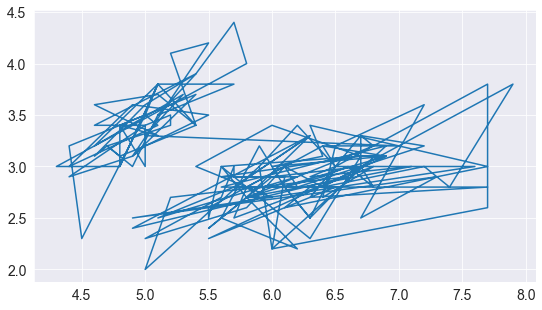The output is not very informative as there are too many combinations of the two properties within the dataset. There doesn't seem to be simple relationship between them.

We can use a scatter plot to visualize how sepal length & sepal width vary using the `scatterplot` function from the `seaborn` module (imported as `sns`).

In :
``sns.scatterplot(x=flowers_df.sepal_length, y=flowers_df.sepal_width);``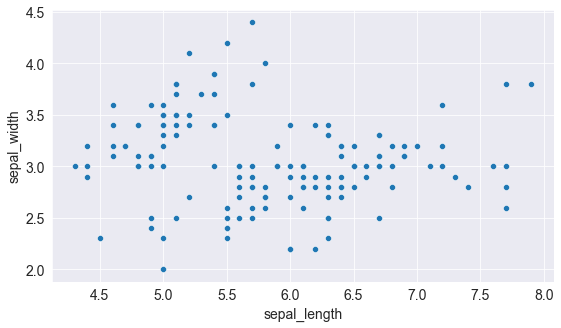Notice how the points in the above plot seem to form distinct clusters with some outliers. We can color the dots using the flower species as a `hue`. We can also make the points larger using the `s` argument.

In :
``sns.scatterplot(x=flowers_df.sepal_length, y=flowers_df.sepal_width, hue=flowers_df.species, s=100);``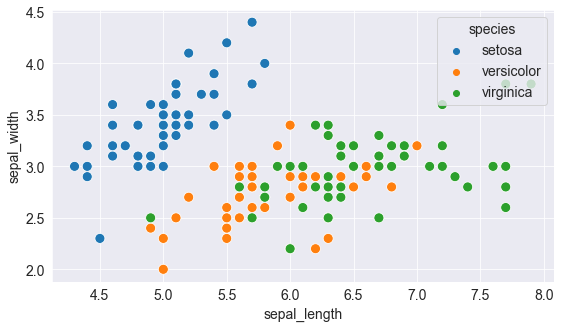Adding hues makes the plot more informative. We can immediately tell that Setosa flowers have a smaller sepal length but higher sepal widths. In contrast, the opposite is true for Virginica flowers.

#### Customizing Seaborn Figures

Since Seaborn uses Matplotlib's plotting functions internally, we can use functions like `plt.figure` and `plt.title` to modify the figure.

In :
``````plt.figure(figsize=(12, 6))
plt.title('Sepal Dimensions')

sns.scatterplot(x=flowers_df.sepal_length,
y=flowers_df.sepal_width,
hue=flowers_df.species,
s=100);``````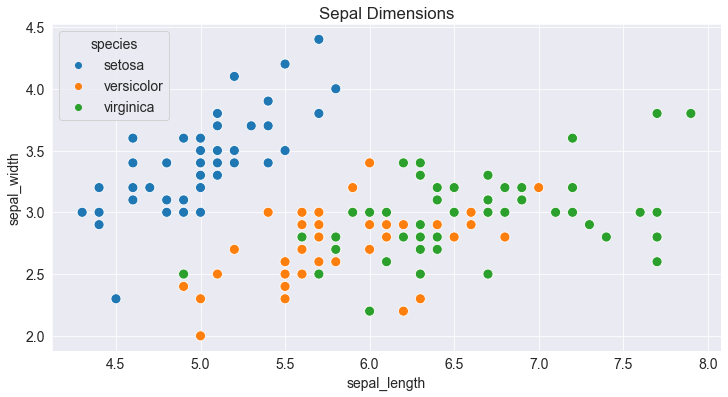#### Plotting using Pandas Data Frames

Seaborn has in-built support for Pandas data frames. Instead of passing each column as a series, you can provide column names and use the `data` argument to specify a data frame.

In :
``````plt.title('Sepal Dimensions')
sns.scatterplot(x='sepal_length',
y='sepal_width',
hue='species',
s=100,
data=flowers_df);
``````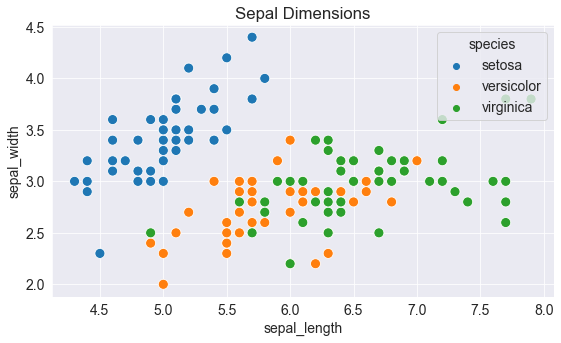Let's save and upload our work before continuing.

In :
``import jovian``
In :
``jovian.commit()``
```[jovian] Attempting to save notebook.. [jovian] Updating notebook "aakashns/python-matplotlib-data-visualization" on https://jovian.ai/ [jovian] Uploading notebook.. [jovian] Capturing environment.. [jovian] Committed successfully! https://jovian.ai/aakashns/python-matplotlib-data-visualization ```
Out:
``'https://jovian.ai/aakashns/python-matplotlib-data-visualization'``

### Histogram

A histogram represents the distribution of a variable by creating bins (interval) along the range of values and showing vertical bars to indicate the number of observations in each bin.

For example, let's visualize the distribution of values of sepal width in the flowers dataset. We can use the `plt.hist` function to create a histogram.

In :
``````# Load data into a Pandas dataframe
In :
``flowers_df.sepal_width``
Out:
``````0      3.5
1      3.0
2      3.2
3      3.1
4      3.6
...
145    3.0
146    2.5
147    3.0
148    3.4
149    3.0
Name: sepal_width, Length: 150, dtype: float64``````
In :
``````plt.title("Distribution of Sepal Width")
plt.hist(flowers_df.sepal_width);
``````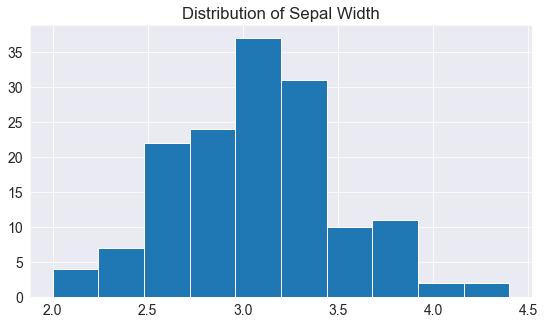We can immediately see that the sepal widths lie in the range 2.0 - 4.5, and around 35 values are in the range 2.9 - 3.1, which seems to be the most populous bin.

#### Controlling the size and number of bins

We can control the number of bins or the size of each one using the bins argument.

In :
``````# Specifying the number of bins
plt.hist(flowers_df.sepal_width, bins=5);``````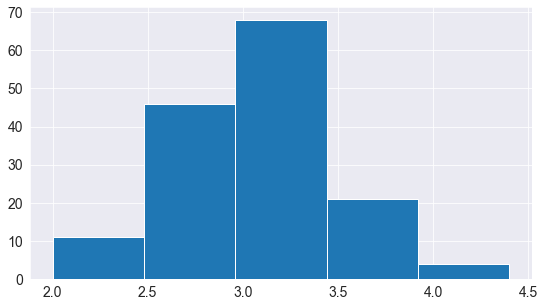In :
``````import numpy as np

# Specifying the boundaries of each bin
plt.hist(flowers_df.sepal_width, bins=np.arange(2, 5, 0.25));``````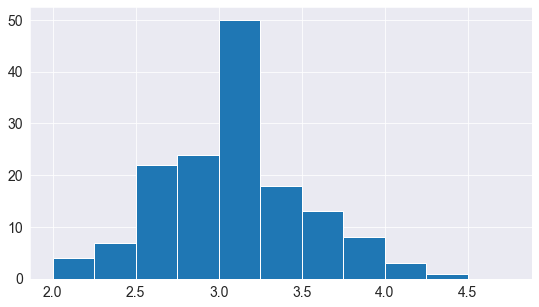In :
``````# Bins of unequal sizes
plt.hist(flowers_df.sepal_width, bins=[1, 3, 4, 4.5]);``````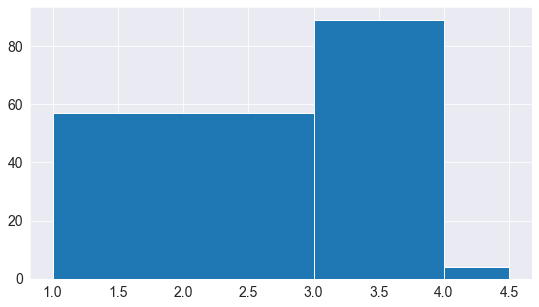#### Multiple Histograms

Similar to line charts, we can draw multiple histograms in a single chart. We can reduce each histogram's opacity so that one histogram's bars don't hide the others'.

Let's draw separate histograms for each species of flowers.

In :
``````setosa_df = flowers_df[flowers_df.species == 'setosa']
versicolor_df = flowers_df[flowers_df.species == 'versicolor']
virginica_df = flowers_df[flowers_df.species == 'virginica']``````
In :
``````plt.hist(setosa_df.sepal_width, alpha=0.4, bins=np.arange(2, 5, 0.25));
plt.hist(versicolor_df.sepal_width, alpha=0.4, bins=np.arange(2, 5, 0.25));``````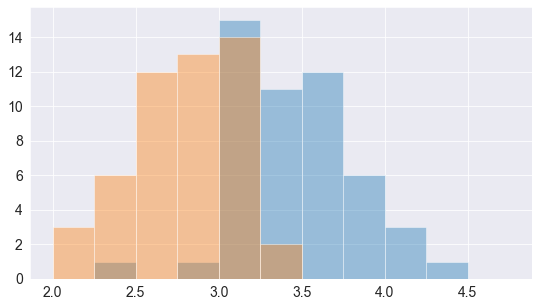We can also stack multiple histograms on top of one another.

In :
``````plt.title('Distribution of Sepal Width')

plt.hist([setosa_df.sepal_width, versicolor_df.sepal_width, virginica_df.sepal_width],
bins=np.arange(2, 5, 0.25),
stacked=True);

plt.legend(['Setosa', 'Versicolor', 'Virginica']);``````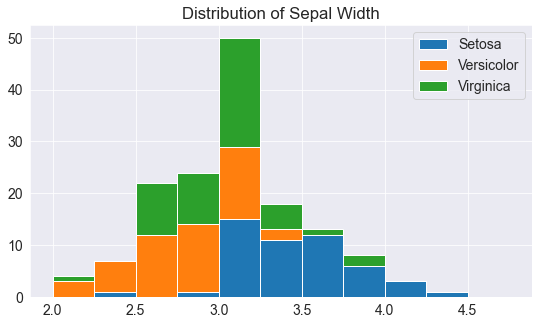Let's save and commit our work before continuing

In :
``import jovian``
In :
``jovian.commit()``
```[jovian] Attempting to save notebook.. [jovian] Updating notebook "aakashns/python-matplotlib-data-visualization" on https://jovian.ai/ [jovian] Uploading notebook.. [jovian] Capturing environment.. [jovian] Committed successfully! https://jovian.ai/aakashns/python-matplotlib-data-visualization ```
Out:
``'https://jovian.ai/aakashns/python-matplotlib-data-visualization'``

### Bar Chart

Bar charts are quite similar to line charts, i.e., they show a sequence of values. However, a bar is shown for each value, rather than points connected by lines. We can use the `plt.bar` function to draw a bar chart.

In :
``````years = range(2000, 2006)
apples = [0.35, 0.6, 0.9, 0.8, 0.65, 0.8]
oranges = [0.4, 0.8, 0.9, 0.7, 0.6, 0.8]``````
In :
``plt.bar(years, oranges);``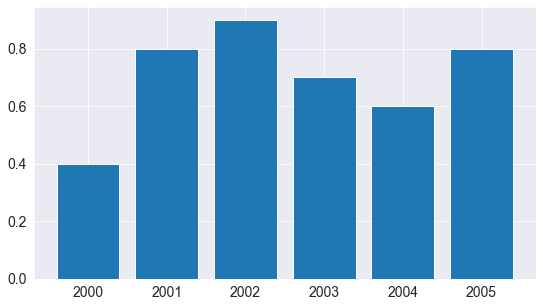Like histograms, we can stack bars on top of one another. We use the `bottom` argument of `plt.bar` to achieve this.

In :
``````plt.bar(years, apples)
plt.bar(years, oranges, bottom=apples);
``````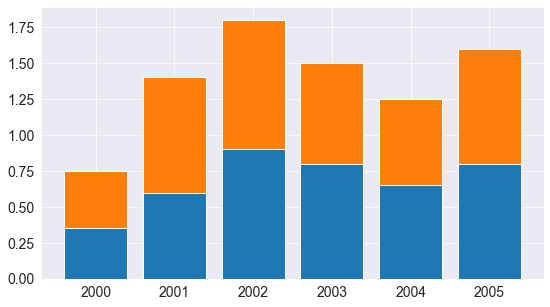#### Bar Plots with Averages

Let's look at another sample dataset included with Seaborn, called `tips`. The dataset contains information about the sex, time of day, total bill, and tip amount for customers visiting a restaurant over a week.

In :
``tips_df = sns.load_dataset("tips");``
In :
``tips_df``
Out:

We might want to draw a bar chart to visualize how the average bill amount varies across different days of the week. One way to do this would be to compute the day-wise averages and then use `plt.bar` (try it as an exercise).

However, since this is a very common use case, the Seaborn library provides a `barplot` function which can automatically compute averages.

In :
``sns.barplot(x='day', y='total_bill', data=tips_df);``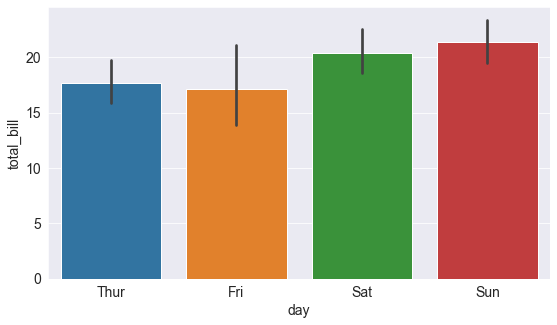The lines cutting each bar represent the amount of variation in the values. For instance, it seems like the variation in the total bill is relatively high on Fridays and low on Saturday.

We can also specify a `hue` argument to compare bar plots side-by-side based on a third feature, e.g., sex.

In :
``sns.barplot(x='day', y='total_bill', hue='sex', data=tips_df);``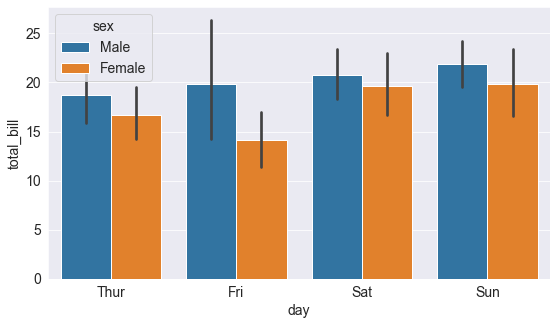You can make the bars horizontal simply by switching the axes.

In :
``sns.barplot(x='total_bill', y='day', hue='sex', data=tips_df);``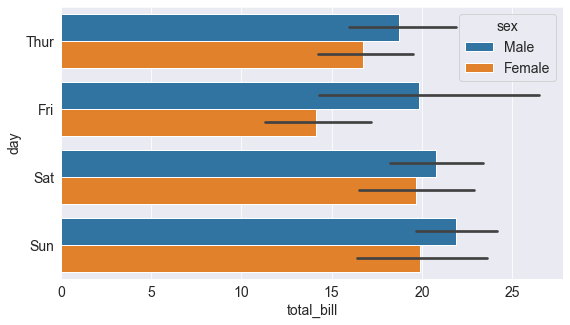Let's save and commit our work before continuing.

In :
``import jovian``
In :
``jovian.commit()``
```[jovian] Attempting to save notebook.. [jovian] Updating notebook "aakashns/python-matplotlib-data-visualization" on https://jovian.ai/ [jovian] Uploading notebook.. [jovian] Capturing environment.. [jovian] Committed successfully! https://jovian.ai/aakashns/python-matplotlib-data-visualization ```
Out:
``'https://jovian.ai/aakashns/python-matplotlib-data-visualization'``

### Heatmap

A heatmap is used to visualize 2-dimensional data like a matrix or a table using colors. The best way to understand it is by looking at an example. We'll use another sample dataset from Seaborn, called `flights`, to visualize monthly passenger footfall at an airport over 12 years.

In :
``flights_df = sns.load_dataset("flights").pivot("month", "year", "passengers")``
In :
``flights_df``
Out:

`flights_df` is a matrix with one row for each month and one column for each year. The values show the number of passengers (in thousands) that visited the airport in a specific month of a year. We can use the `sns.heatmap` function to visualize the footfall at the airport.

In :
``````plt.title("No. of Passengers (1000s)")
sns.heatmap(flights_df);
``````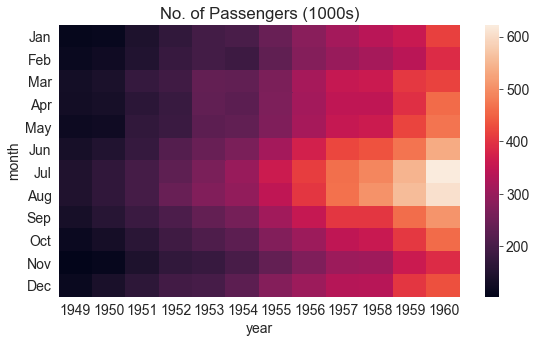The brighter colors indicate a higher footfall at the airport. By looking at the graph, we can infer two things:

• The footfall at the airport in any given year tends to be the highest around July & August.
• The footfall at the airport in any given month tends to grow year by year.

We can also display the actual values in each block by specifying `annot=True` and using the `cmap` argument to change the color palette.

In :
``````plt.title("No. of Passengers (1000s)")
sns.heatmap(flights_df, fmt="d", annot=True, cmap='Blues');``````